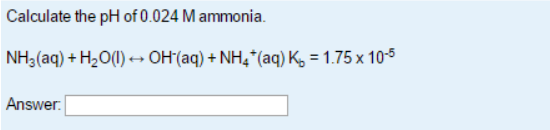# Problem: Calculate the pH of 0.024 M ammonia. NH3 (aq) + H2O (l) &lt;-&gt;  OH - (aq) + NH4+(aq)     K b = 1.75 x 10 -5

###### FREE Expert Solution
91% (271 ratings)###### Problem Details

Calculate the pH of 0.024 M ammonia.

NH3 (aq) + H2O (l) <->  OH (aq) + NH4+(aq)     K b = 1.75 x 10 -5